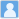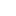• ### Thermodynamic properties at the kinetic freeze-out in the Au+Au and Cu+Cu collisions at the RHIC

分类： 物理学 >> 核物理学 提交时间： 2023-09-07Wu, Weihao Tao, Junqi Zheng, Hua Zhang, Wenchao Liu, Xingquan Zhu, Lilin Bonasera, Aldo

摘要：The thermodynamic properties of charged particles, such as the energy density, pressure, entropy density, particle density, and squared speed of sound at the kinetic freeze-out in the $mathrm{Au+Au}$ collisions from the relativistic heavy ion collider (RHIC) beam energy scan program ($sqrt{s_ text{NN}}$=7.7--200 GeV) and in the $mathrm{Cu+Cu}$ collisions at $sqrt{s_ text{NN}}$=62.4, 200 GeV are studied using the thermodynamically consistent Tsallis distribution. The energy density, pressure, and particle density decrease monotonically with the collision energy for the same collision centrality; These properties also decrease monotonically from the central to peripheral collisions at the same collision energy. While the scaled energy density $varepsilon/T^4$ and scaled entropy density $s/T^3$ demonstrate the opposite trend with the collision energy for the same collision centrality. There is a correlation between $varepsilon/T^4$ and $s/T^3$ at the same centrality. In addition, the squared speed of sound was calculated to determine that all the collision energies share nearly the same value at different collision centralities.通过